# Mean State

Period Mean (original grids) [W/m2]
Model Period Mean (intersection) [W/m2]
Model Period Mean (complement) [W/m2]
Benchmark Period Mean (intersection) [W/m2]
Benchmark Period Mean (complement) [W/m2]
Bias [W/m2]
RMSE [W/m2]
Phase Shift [months]
Bias Score 
RMSE Score 
Seasonal Cycle Score 
Spatial Distribution Score 
Interannual Variability Score 
Overall Score 
Benchmark [-] 457.
CLM4 [-] 458. 458. 0.00 456. 465. 2.39 14.9 1.26 0.47 0.38 0.82 0.94 0.63 0.60
CLM4.5 [-] 462. 462. 0.00 456. 465. 6.40 16.3 1.35 0.43 0.38 0.81 0.96 0.64 0.60
CLM5 [-] 463. 463. 0.00 456. 465. 7.83 16.4 1.44 0.46 0.38 0.80 0.98 0.61 0.60
Period Mean (original grids) [W/m2]
Model Period Mean (intersection) [W/m2]
Model Period Mean (complement) [W/m2]
Benchmark Period Mean (intersection) [W/m2]
Benchmark Period Mean (complement) [W/m2]
Bias [W/m2]
RMSE [W/m2]
Phase Shift [months]
Bias Score 
RMSE Score 
Seasonal Cycle Score 
Spatial Distribution Score 
Interannual Variability Score 
Overall Score 
Benchmark [-] 466.
CLM4 [-] 476. 477. 0.00 467. 458. 10.1 19.4 0.592 0.59 0.50 0.95 1.0 0.67 0.70
CLM4.5 [-] 482. 482. 0.00 467. 458. 15.3 22.2 0.529 0.49 0.51 0.95 0.94 0.65 0.68
CLM5 [-] 484. 484. 0.00 467. 458. 17.2 23.5 0.637 0.46 0.50 0.94 0.95 0.62 0.66
Period Mean (original grids) [W/m2]
Model Period Mean (intersection) [W/m2]
Model Period Mean (complement) [W/m2]
Benchmark Period Mean (intersection) [W/m2]
Benchmark Period Mean (complement) [W/m2]
Bias [W/m2]
RMSE [W/m2]
Phase Shift [months]
Bias Score 
RMSE Score 
Seasonal Cycle Score 
Spatial Distribution Score 
Interannual Variability Score 
Overall Score 
Benchmark [-] 443.
CLM4 [-] 446. 446. 0.00 443. 446. 4.18 16.7 1.08 0.64 0.48 0.86 1.0 0.66 0.69
CLM4.5 [-] 453. 453. 0.00 443. 446. 9.71 18.3 1.09 0.56 0.49 0.86 1.0 0.66 0.67
CLM5 [-] 452. 452. 0.00 443. 446. 9.13 18.8 1.38 0.57 0.46 0.81 1.0 0.60 0.65
Period Mean (original grids) [W/m2]
Model Period Mean (intersection) [W/m2]
Model Period Mean (complement) [W/m2]
Benchmark Period Mean (intersection) [W/m2]
Benchmark Period Mean (complement) [W/m2]
Bias [W/m2]
RMSE [W/m2]
Phase Shift [months]
Bias Score 
RMSE Score 
Seasonal Cycle Score 
Spatial Distribution Score 
Interannual Variability Score 
Overall Score 
Benchmark [-] 279.
CLM4 [-] 276. 276. 0.00 278. 296. 0.256 15.9 0.121 0.91 0.80 0.99 0.99 0.78 0.88
CLM4.5 [-] 275. 276. 0.00 278. 296. 0.179 15.2 0.123 0.91 0.81 0.99 1.0 0.76 0.88
CLM5 [-] 277. 278. 0.00 278. 296. 2.37 15.4 0.127 0.90 0.81 0.99 1.0 0.74 0.88
Period Mean (original grids) [W/m2]
Model Period Mean (intersection) [W/m2]
Model Period Mean (complement) [W/m2]
Benchmark Period Mean (intersection) [W/m2]
Benchmark Period Mean (complement) [W/m2]
Bias [W/m2]
RMSE [W/m2]
Phase Shift [months]
Bias Score 
RMSE Score 
Seasonal Cycle Score 
Spatial Distribution Score 
Interannual Variability Score 
Overall Score 
Benchmark [-] 457.
CLM4 [-] 452. 452. 0.00 454. 467. -0.662 13.6 1.63 0.37 0.37 0.76 0.59 0.60 0.51
CLM4.5 [-] 454. 454. 0.00 454. 467. 0.984 14.0 1.68 0.33 0.38 0.74 0.60 0.59 0.50
CLM5 [-] 459. 459. 0.00 454. 467. 5.54 14.6 1.55 0.29 0.38 0.77 0.65 0.58 0.51
Period Mean (original grids) [W/m2]
Model Period Mean (intersection) [W/m2]
Model Period Mean (complement) [W/m2]
Benchmark Period Mean (intersection) [W/m2]
Benchmark Period Mean (complement) [W/m2]
Bias [W/m2]
RMSE [W/m2]
Phase Shift [months]
Bias Score 
RMSE Score 
Seasonal Cycle Score 
Spatial Distribution Score 
Interannual Variability Score 
Overall Score 
Benchmark [-] 312.
CLM4 [-] 313. 313. 0.00 311. 334. 2.07 10.5 0.0356 0.94 0.88 1.0 1.0 0.88 0.93
CLM4.5 [-] 314. 314. 0.00 311. 334. 2.37 10.6 0.0385 0.94 0.88 1.0 1.0 0.87 0.93
CLM5 [-] 317. 317. 0.00 311. 334. 5.51 11.6 0.0521 0.91 0.88 1.0 1.0 0.86 0.92
Period Mean (original grids) [W/m2]
Model Period Mean (intersection) [W/m2]
Model Period Mean (complement) [W/m2]
Benchmark Period Mean (intersection) [W/m2]
Benchmark Period Mean (complement) [W/m2]
Bias [W/m2]
RMSE [W/m2]
Phase Shift [months]
Bias Score 
RMSE Score 
Seasonal Cycle Score 
Spatial Distribution Score 
Interannual Variability Score 
Overall Score 
Benchmark [-] 364.
CLM4 [-] 362. 361. 0.00 363. 399. 0.496 13.2 0.107 0.87 0.83 0.99 0.99 0.78 0.88
CLM4.5 [-] 362. 362. 0.00 363. 399. 0.962 13.2 0.105 0.87 0.83 0.99 0.99 0.78 0.88
CLM5 [-] 366. 365. 0.00 363. 399. 4.07 14.2 0.164 0.86 0.82 0.99 1.0 0.76 0.87
Period Mean (original grids) [W/m2]
Model Period Mean (intersection) [W/m2]
Model Period Mean (complement) [W/m2]
Benchmark Period Mean (intersection) [W/m2]
Benchmark Period Mean (complement) [W/m2]
Bias [W/m2]
RMSE [W/m2]
Phase Shift [months]
Bias Score 
RMSE Score 
Seasonal Cycle Score 
Spatial Distribution Score 
Interannual Variability Score 
Overall Score 
Benchmark [-] 448.
CLM4 [-] 447. 448. 0.00 446. 467. 3.72 16.5 0.643 0.66 0.56 0.94 0.99 0.68 0.73
CLM4.5 [-] 452. 452. 0.00 446. 467. 7.61 18.1 0.659 0.61 0.57 0.93 0.99 0.67 0.72
CLM5 [-] 453. 454. 0.00 446. 467. 9.50 18.9 0.825 0.60 0.56 0.91 0.98 0.63 0.71
Period Mean (original grids) [W/m2]
Model Period Mean (intersection) [W/m2]
Model Period Mean (complement) [W/m2]
Benchmark Period Mean (intersection) [W/m2]
Benchmark Period Mean (complement) [W/m2]
Bias [W/m2]
RMSE [W/m2]
Phase Shift [months]
Bias Score 
RMSE Score 
Seasonal Cycle Score 
Spatial Distribution Score 
Interannual Variability Score 
Overall Score 
Benchmark [-] 281.
CLM4 [-] 282. 282. 0.00 281. 288. 2.23 14.4 0.0850 0.93 0.80 0.99 1.0 0.79 0.89
CLM4.5 [-] 281. 282. 0.00 281. 288. 1.72 12.7 0.0824 0.94 0.82 0.99 1.0 0.77 0.89
CLM5 [-] 284. 284. 0.00 281. 288. 4.17 13.2 0.0840 0.91 0.83 0.99 1.0 0.77 0.89
Period Mean (original grids) [W/m2]
Model Period Mean (intersection) [W/m2]
Model Period Mean (complement) [W/m2]
Benchmark Period Mean (intersection) [W/m2]
Benchmark Period Mean (complement) [W/m2]
Bias [W/m2]
RMSE [W/m2]
Phase Shift [months]
Bias Score 
RMSE Score 
Seasonal Cycle Score 
Spatial Distribution Score 
Interannual Variability Score 
Overall Score 
Benchmark [-] 441.
CLM4 [-] 447. 447. 0.00 443. 426. 1.41 12.4 0.347 0.81 0.70 0.97 0.99 0.78 0.83
CLM4.5 [-] 447. 447. 0.00 443. 426. 1.93 12.8 0.354 0.80 0.70 0.97 0.99 0.78 0.82
CLM5 [-] 449. 449. 0.00 443. 426. 3.62 13.2 0.381 0.80 0.69 0.97 0.99 0.74 0.81
Period Mean (original grids) [W/m2]
Model Period Mean (intersection) [W/m2]
Model Period Mean (complement) [W/m2]
Benchmark Period Mean (intersection) [W/m2]
Benchmark Period Mean (complement) [W/m2]
Bias [W/m2]
RMSE [W/m2]
Phase Shift [months]
Bias Score 
RMSE Score 
Seasonal Cycle Score 
Spatial Distribution Score 
Interannual Variability Score 
Overall Score 
Benchmark [-] 368.
CLM4 [-] 362. 362. 0.00 366. 389. -1.28 12.2 0.248 0.85 0.76 0.98 1.0 0.81 0.86
CLM4.5 [-] 361. 361. 0.00 366. 389. -1.80 11.7 0.207 0.84 0.77 0.99 1.0 0.81 0.86
CLM5 [-] 365. 365. 0.00 366. 389. 1.41 11.8 0.429 0.86 0.77 0.97 1.0 0.78 0.86
Period Mean (original grids) [W/m2]
Model Period Mean (intersection) [W/m2]
Model Period Mean (complement) [W/m2]
Benchmark Period Mean (intersection) [W/m2]
Benchmark Period Mean (complement) [W/m2]
Bias [W/m2]
RMSE [W/m2]
Phase Shift [months]
Bias Score 
RMSE Score 
Seasonal Cycle Score 
Spatial Distribution Score 
Interannual Variability Score 
Overall Score 
Benchmark [-] 399.
CLM4 [-] 377. 377. 0.00 376. 409. 2.37 14.8 0.497 0.76 0.67 0.94 1.0 0.73 0.79
CLM4.5 [-] 378. 378. 0.00 376. 409. 3.73 15.1 0.495 0.74 0.67 0.94 1.0 0.72 0.79
CLM5 [-] 381. 381. 0.00 376. 409. 6.29 16.1 0.539 0.72 0.67 0.94 1.0 0.69 0.78
Period Mean (original grids) [W/m2]
Model Period Mean (intersection) [W/m2]
Model Period Mean (complement) [W/m2]
Benchmark Period Mean (intersection) [W/m2]
Benchmark Period Mean (complement) [W/m2]
Bias [W/m2]
RMSE [W/m2]
Phase Shift [months]
Bias Score 
RMSE Score 
Seasonal Cycle Score 
Spatial Distribution Score 
Interannual Variability Score 
Overall Score 
Benchmark [-] 450.
CLM4 [-] 451. 450. 0.00 450. 446. 0.525 14.7 0.346 0.85 0.76 0.97 1.0 0.74 0.85
CLM4.5 [-] 451. 451. 0.00 450. 446. 0.875 14.7 0.336 0.85 0.76 0.97 1.0 0.74 0.85
CLM5 [-] 452. 452. 0.00 450. 446. 2.24 15.1 0.358 0.84 0.76 0.97 1.0 0.72 0.84
Period Mean (original grids) [W/m2]
Model Period Mean (intersection) [W/m2]
Model Period Mean (complement) [W/m2]
Benchmark Period Mean (intersection) [W/m2]
Benchmark Period Mean (complement) [W/m2]
Bias [W/m2]
RMSE [W/m2]
Phase Shift [months]
Bias Score 
RMSE Score 
Seasonal Cycle Score 
Spatial Distribution Score 
Interannual Variability Score 
Overall Score 
Benchmark [-] 281.
CLM4 [-] 284. 284. 0.00 281. 293. 3.30 14.8 0.172 0.91 0.80 0.99 1.0 0.80 0.88
CLM4.5 [-] 284. 284. 0.00 281. 293. 3.03 14.9 0.160 0.91 0.80 0.99 1.0 0.78 0.88
CLM5 [-] 287. 286. 0.00 281. 293. 6.06 16.8 0.168 0.89 0.79 0.99 1.0 0.76 0.87
Period Mean (original grids) [W/m2]
Model Period Mean (intersection) [W/m2]
Model Period Mean (complement) [W/m2]
Benchmark Period Mean (intersection) [W/m2]
Benchmark Period Mean (complement) [W/m2]
Bias [W/m2]
RMSE [W/m2]
Phase Shift [months]
Bias Score 
RMSE Score 
Seasonal Cycle Score 
Spatial Distribution Score 
Interannual Variability Score 
Overall Score 
Benchmark [-] 442.
CLM4 [-] 446. 446. 0.00 439. 459. 11.0 18.4 0.860 0.52 0.55 0.89 0.99 0.73 0.71
CLM4.5 [-] 446. 446. 0.00 439. 459. 10.4 18.3 0.873 0.52 0.55 0.89 0.97 0.72 0.70
CLM5 [-] 447. 447. 0.00 439. 459. 12.0 18.6 0.885 0.51 0.56 0.89 0.95 0.67 0.69
Period Mean (original grids) [W/m2]
Model Period Mean (intersection) [W/m2]
Model Period Mean (complement) [W/m2]
Benchmark Period Mean (intersection) [W/m2]
Benchmark Period Mean (complement) [W/m2]
Bias [W/m2]
RMSE [W/m2]
Phase Shift [months]
Bias Score 
RMSE Score 
Seasonal Cycle Score 
Spatial Distribution Score 
Interannual Variability Score 
Overall Score 
Benchmark [-] 386.
CLM4 [-] 385. 385. 0.00 384. 429. 3.03 9.72 0.120 0.90 0.85 0.99 1.0 0.88 0.91
CLM4.5 [-] 387. 387. 0.00 384. 429. 4.69 10.5 0.0936 0.88 0.85 0.99 1.0 0.88 0.91
CLM5 [-] 391. 391. 0.00 384. 429. 8.89 13.0 0.112 0.82 0.86 0.99 0.99 0.85 0.90
Period Mean (original grids) [W/m2]
Model Period Mean (intersection) [W/m2]
Model Period Mean (complement) [W/m2]
Benchmark Period Mean (intersection) [W/m2]
Benchmark Period Mean (complement) [W/m2]
Bias [W/m2]
RMSE [W/m2]
Phase Shift [months]
Bias Score 
RMSE Score 
Seasonal Cycle Score 
Spatial Distribution Score 
Interannual Variability Score 
Overall Score 
Benchmark [-] 324.
CLM4 [-] 325. 325. 0.00 323. 347. 2.59 11.7 0.0506 0.91 0.83 1.0 0.98 0.80 0.89
CLM4.5 [-] 325. 325. 0.00 323. 347. 2.59 11.8 0.0528 0.91 0.83 1.0 0.98 0.79 0.89
CLM5 [-] 328. 328. 0.00 323. 347. 5.87 13.2 0.104 0.88 0.83 0.99 0.97 0.78 0.88
Period Mean (original grids) [W/m2]
Model Period Mean (intersection) [W/m2]
Model Period Mean (complement) [W/m2]
Benchmark Period Mean (intersection) [W/m2]
Benchmark Period Mean (complement) [W/m2]
Bias [W/m2]
RMSE [W/m2]
Phase Shift [months]
Bias Score 
RMSE Score 
Seasonal Cycle Score 
Spatial Distribution Score 
Interannual Variability Score 
Overall Score 
Benchmark [-] 377.
CLM4 [-] 378. 379. 0.00 377. 378. 2.19 12.8 0.0869 0.86 0.82 0.99 1.0 0.78 0.88
CLM4.5 [-] 378. 380. 0.00 377. 378. 2.51 12.8 0.0852 0.86 0.82 0.99 1.0 0.79 0.88
CLM5 [-] 381. 382. 0.00 377. 378. 5.35 14.1 0.110 0.84 0.81 0.99 1.0 0.74 0.87
Period Mean (original grids) [W/m2]
Model Period Mean (intersection) [W/m2]
Model Period Mean (complement) [W/m2]
Benchmark Period Mean (intersection) [W/m2]
Benchmark Period Mean (complement) [W/m2]
Bias [W/m2]
RMSE [W/m2]
Phase Shift [months]
Bias Score 
RMSE Score 
Seasonal Cycle Score 
Spatial Distribution Score 
Interannual Variability Score 
Overall Score 
Benchmark [-] 449.
CLM4 [-] 453. 453. 0.00 449. 463. 4.81 12.6 1.19 0.44 0.39 0.84 0.98 0.65 0.62
CLM4.5 [-] 456. 456. 0.00 449. 463. 7.39 14.0 1.15 0.36 0.39 0.85 0.97 0.65 0.60
CLM5 [-] 458. 458. 0.00 449. 463. 9.09 14.8 1.21 0.32 0.40 0.84 0.99 0.63 0.59
Period Mean (original grids) [W/m2]
Model Period Mean (intersection) [W/m2]
Model Period Mean (complement) [W/m2]
Benchmark Period Mean (intersection) [W/m2]
Benchmark Period Mean (complement) [W/m2]
Bias [W/m2]
RMSE [W/m2]
Phase Shift [months]
Bias Score 
RMSE Score 
Seasonal Cycle Score 
Spatial Distribution Score 
Interannual Variability Score 
Overall Score 
Benchmark [-] 443.
CLM4 [-] 445. 444. 0.00 443. 434. 0.689 14.3 0.848 0.71 0.55 0.88 0.98 0.70 0.73
CLM4.5 [-] 447. 447. 0.00 443. 434. 3.04 14.5 0.944 0.68 0.56 0.86 0.98 0.71 0.73
CLM5 [-] 449. 449. 0.00 443. 434. 5.44 14.2 0.895 0.70 0.56 0.87 0.99 0.66 0.72
Period Mean (original grids) [W/m2]
Model Period Mean (intersection) [W/m2]
Model Period Mean (complement) [W/m2]
Benchmark Period Mean (intersection) [W/m2]
Benchmark Period Mean (complement) [W/m2]
Bias [W/m2]
RMSE [W/m2]
Phase Shift [months]
Bias Score 
RMSE Score 
Seasonal Cycle Score 
Spatial Distribution Score 
Interannual Variability Score 
Overall Score 
Benchmark [-] 383.
CLM4 [-] 384. 383. 0.00 381. 396. 2.84 19.9 0.905 0.64 0.57 0.89 1.0 0.66 0.72
CLM4.5 [-] 386. 385. 0.00 381. 396. 4.47 20.2 0.914 0.63 0.57 0.89 1.0 0.66 0.72
CLM5 [-] 387. 386. 0.00 381. 396. 5.53 20.2 0.891 0.63 0.57 0.89 1.0 0.64 0.72

# Temporally integrated period mean

BENCHMARK MEAN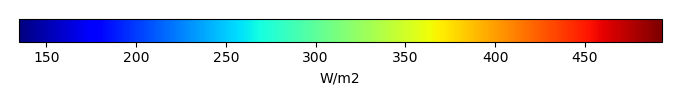MODEL MEANBIAS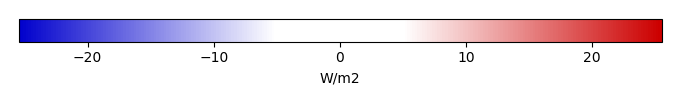BIAS SCORERMSE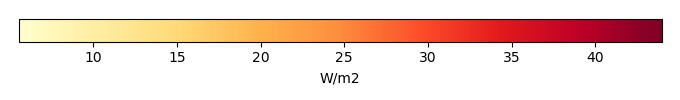RMSE SCOREBENCHMARK INTERANNUAL VARIABILITY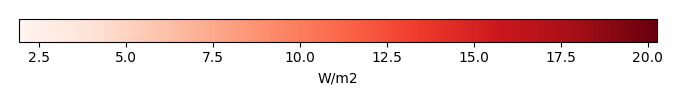MODEL INTERANNUAL VARIABILITYINTERANNUAL VARIABILITY SCOREBENCHMARK MAX MONTHMODEL MAX MONTHDIFFERENCE IN MAX MONTH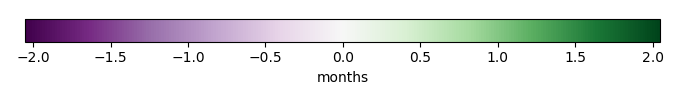SEASONAL CYCLE SCORESPATIAL TAYLOR DIAGRAMMODEL COLORS# Spatially integrated regional mean

MODEL COLORSREGIONAL MEANANNUAL CYCLEMONTHLY ANOMALYANNUAL CYCLE# All Models

BenchmarkCLM4CLM4.5CLM5# Data Information

creation_date: Thu May 8 23:13:11 PDT 2014

source_file: This product is generated from monthly 1 degree CERES EBAF Radiation observations